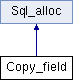My Project
Copy_field Class Reference
Inheritance diagram for Copy_field:List of all members.

## Public Member Functions

void set (Field *to, Field *from, bool save)
void set (uchar *to, Field *from)

## Public Attributes

uchar * from_ptr
uchar * to_ptr
uchar * from_null_ptr
uchar * to_null_ptr
my_bool * null_row
uint from_bit
uint to_bit
uint from_length
uint to_length
Fieldfrom_field
Fieldto_field
String tmp
void(* do_copy )(Copy_field *)
void(* do_copy2 )(Copy_field *)

## Member Function Documentation

 void Copy_field::set ( uchar * to, Field * from )

copy of field to maybe null string. If field is null then the all bytes are set to 0. if field is not null then the first byte is set to 1 and the rest of the string is the field value. The 'to' buffer should have a size of field->pack_length()+1

## Member Data Documentation

Number of bytes in the fields pointed to by 'from_ptr' and 'to_ptr'. Usually this is the number of bytes that are copied from 'from_ptr' to 'to_ptr'.

For variable-length fields (VARCHAR), the first byte(s) describe the actual length of the text. For VARCHARs with length < 256 there is 1 length byte >= 256 there is 2 length bytes Thus, if from_field is VARCHAR(10), from_length (and in most cases to_length) is 11. For VARCHAR(1024), the length is 1026.